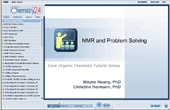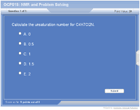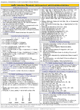How to Learn in 24 Hours?The Rapid Learning Movie

 Need Help? M-F: 9am-5pm(PST): Toll-Free: (877) RAPID-10 US Direct: (714) 692-2900 Int'l: 001-714-692-2900 24/7 Online Technical Support: The Rapid Support Center Secure Online Order:Got Questions? Frequently Asked Questions
 Need Proof? Testimonials by Our Users
 Trustlink is a Better Business Bureau Program. Rapid Learning Center is a fivr-star business. External TrustLink Reviews

 Member Login: User ID: Password:

 Rapid Learning Courses: MCAT in 24 Hours (2015-16) USMLE in 24 Hours (Boards) Chemistry in 24 Hours Biology in 24 Hours Physics in 24 Hours Mathematics in 24 Hours Psychology in 24 Hours SAT in 24 Hours ACT in 24 Hours AP in 24 Hours CLEP in 24 Hours DAT in 24 Hours (Dental) OAT in 24 Hours (Optometry) PCAT in 24 Hours (Pharmacy) Nursing Entrance Exams Certification in 24 Hours eBook - Survival Kits Audiobooks (MP3)

 Tell-A-Friend: Have friends taking science and math courses too? Tell them about our rapid learning system.Home »  Chemistry »  Organic Chemistry

NMR and Problem Solving

 Topic Review on "Title": NMR Background Method of structure determination based upon the relative positions of hydrogens and carbons in the spectra.  Only nuclei with an odd number of neutrons or an odd number of protons can give rise to an NMR signal.  The most common nuclei are 1H and 13C. NMR Theory A compound is placed in a magnetic field.  The nuclei align themselves either with or against the magnetic field.  With the right combination of magnetic field and electromagnetic radiation, the nuclei flips its spin.  The absorption of energy is detected by the NMR spectrometer. NMR Definitions Chemical shift is the difference, in ppm, between the frequency of TMS and the frequency of the observed atom.  TMS, tetramethylsilane [(CH3)4Si], is an internal standard that has a chemical shift of δ 0.00.  Integration is the area under a peak that is proportional to the number of hydrogens on adjacent carbons.  Spin-spin coupling is the splitting of signals into multiplets when the magnetic field of the proton is affected by protons on adjacent carbons.  Coupling constant is the distance between the peaks of a multiplet. Unsaturation Number U  =  C  +  1  -  2H  +  2N.   U = unsaturation number.  C = number of carbons.  H = number of hydrogens + halogens.  N = number of nitrogens + phosphorus.  The interpretation of unsaturation number follows.  U = 0; no double bonds, triple bond, or rings.  U = 1; 1 double bond or ring.  U = 2; 2 double bonds, 2 rings, 1 triple bond, or 1 double bond + 1 ring.  U = 3; 3 double bonds, 3 rings, 1 double bond + 2 rings, 2 double bonds + 1 ring, 1 triple bond + 1 double bond, or 1 triple bond + 1 ring.  U = 4; benzene.  U = 5 (4 + 1); benzene + 1 double bond or ring.  U = 6 (4 + 2); benzene + 2 double bonds or 2 rings or 1 triple bond or 1 double bond + 1 ring. 1H NMR Spectroscopy The number of signals is equal to the number of different types of protons.  Chemical shift is the electronic structure close to a proton.  Intensities of signals corresponds to the number of protons attached to that carbon.  Splitting of signals is the number of protons on adjacent carbons. 1H NMR Spectroscopy B Splitting of Signals Singlet, s (one peak) = 0 H on adjacent C.  Doublet, d (two peaks, 1:1) = 1 H on adjacent C.  Triplet, t (three peaks, 1:2:1) = 2 H on adjacent C.  Quartet, q (four peaks, 1:3:3:1) = 3 H on adjacent C.  Quintet, quint (five peaks, 1:4:6:4:1) = 4 H on adjacent C.  Sextet, sext (six peaks, 1:5:10:10:5:1) = 5 H on adjacent C. Septet, sept (seven peaks, 1:5:10:10:5:1) = 5 H on adjacent C. Multiplet, m (many peaks) = cannot tell number of H. Proton Magnetic Resonance Frequencies The proton magnetic resonance frequencies are given for aldehyde, vinylic, aromatic, acetylenic, alcohol, carboxylic acid, phenolic, amino, and amide. 1H NMR Problem Solving Calculate the unsaturation number and give the interpretation.  List the chemical shifts.  Compare to Proton Magnetic Resonance tables.  List the splitting.  Measure the vertical distance of integration with ruler.   Add up integrations.  Determine multiplication factor by dividing H by sum of integrations. Multiply each integration by multiplication factor to get H per signal.  For interpretation, use calculated H that are adjacent to splitting minus 1.  Put the structure together, following the formula.  Examples are given. 13C NMR Spectroscopy The number of signals is equal to the number of different types of carbons.  Chemical shift is the type of functional group for that carbon.  Peak areas corresponds to the number of carbons.  The splitting of signals (can also be determined by DEPT, another NMR method) is interpreted as q = CH3; t = CH2; d = CH; s = C. Carbon Magnetic Resonance Frequencies The carbon magnetic resonance frequencies are given for the carbon in alkyl, alkyl fluoride, alkyl chloride, alkyl bromide, alkyl iodide, amine, alcohol, ether, ester, alkyne, alkene, aromatic, nitrile, amide, carboxylic acid, ester, anhydride, anhydride, aldehyde, and ketone. 13C NMR Problem Solving Calculate the unsaturation number and give interpretation.  List the chemical shifts.  List the splitting.  Give the interpretation of the splitting.  Give the interpretation by chemical shift, by referring to the Carbon Magnetic Resonance Frequency tables.  Put the structure together.  Examples are given.

Rapid Study Kit for "Title":
 Flash Movie Flash Game Flash Card Core Concept Tutorial Problem Solving Drill Review Cheat Sheet"Title" Tutorial Summary : The basic information for NMR includes background, theory, definitions, and unsaturation number.  Proton nuclear magnetic resonance spectroscopy is described by background information, splitting of signals, proton resonance frequencies table, problem solving, and examples.  Carbon-13 nuclear resonance spectroscopy can be described by the background information, the carbon magnetic resonance frequencies, problem solving, and examples.

 Tutorial Features: This tutorial provides the comprehensive coverage of the chapter with easy introduction and simple illustration. It features: Concept map showing interconnections of new concepts in this tutorial and those previously introduced. Definition slides introduce terms as they are needed. Visual representation of concepts. Use of colors to emphasis points. Outline of NMR characteristics. Detailed nuclear magnetic resonance tables. Examples of spectra worked out step-by-step. Description of problem solving steps. A concise summary is given at the conclusion of the tutorial.

 "Title" Topic List: Basic Information for NMR Background Theory Definitions Unsaturation Number 1H NMR Spectroscopy Background Information Splitting of Signals Proton Resonance Frequencies Table Problem Solving Examples 13C NMR Spectroscopy Background Information Carbon Magnetic Resonance Frequencies Problem Solving Example

See all 24 lessons in college chemistry, including concept tutorials, problem drills and cheat sheets:
Teach Yourself Organic Chemistry Visually in 24 Hours© 2016 Rapid Learning Inc. All rights reserved         Disclaimer | Privacy Policy | Affiliates